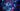# Merge Sort: Trading Space for Time

June 7, 2021

·

DS&A Adventures

·The sorting algorithms that we have reviewed so far - selection, insertion, shell - had constant space complexity, meaning they used a fixed amount of memory that was not dependent on the size of the input. The algorithm we consider in this post, the merge sort, reaches the limit of efficiency but at the cost of additional space.

The merge sort proceeds by using the divide and conquer method. Namely, it splits a list into two sub-lists, recursively down to the base case of 1, and sorts each half. On the way back up the recursion, it merges the halves together again. The merge itself simply picks the lowest number from each half to put in place until entries are exhausted (and so on).

The recursive `sort` method, in the form of a local lambda, looks like this.

``````std::function<void(int, int)> sort = [&combine, &sort](int low, int high)
{
if (high <= low) // base case
{
return;
}

int mid = low + (high - low) / 2;
sort(low, mid); // sort left half
sort(mid + 1, high); // sort right half
combine(low, mid, high); // combine both halves
};``````

Provided a given `low` and `high` index, we first calculate the `mid` index, which marks the middle of the current list. We then sort the left and right halves of the current list, and then `combine` the halves. The `combine` function is where the magic happens.

``````auto combine = [&list, &aux](int low, int mid, int high)
{
// populate aux with relevant elements
for (int i = low; i <= high; ++i)
{
aux[i] = list[i];
}

// set pointers for left and right lists
int left = low;
int right = mid + 1;

// go across range, picking least as we go
for (int i = low; i <= high; ++i)
{
if (left > mid) // ran out of left
{
list[i] = aux[right++];
}

else if (right > high) // ran out of right
{
list[i] = aux[left++];
}

else if (aux[right] < aux[left]) // right smaller
{
list[i] = aux[right++];
}

else // left smaller
{
list[i] = aux[left++];
}
}
};``````

Using two pointers to represent each half, `left` and `right`, we store the unsorted values for each half in the temporary `aux` array and then overwrite the original list with sorted values, picking the lowest value from both halves as we go until we have reached the end of both halves. Thus, by the time we reach the top of the recursion, the full list will have been sorted. To begin the recursion, we call the `sort` method for the full list.

``sort(0, list.size() - 1);``

This algorithm achieves linearithmic complexity (O(nlogn)), which represents optimal efficiency for sorting algorithms. However, the tradeoff is that we must maintain the `aux` list, which takes space proportional to the size of the input. Thus, the merge sort may not be the best choice for memory-bound applications.